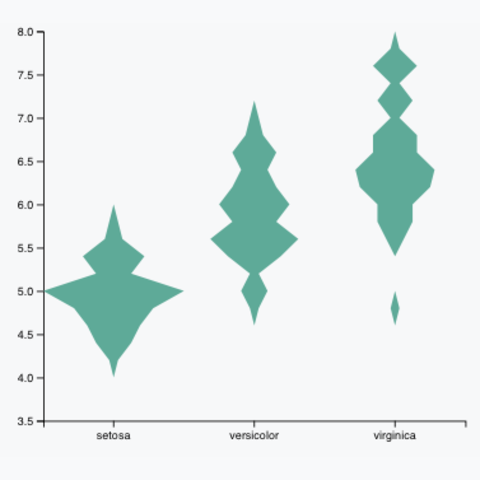# Most basic violin plot in d3.js

A violin plot is an alternative to the more usual boxplot, especially interesting with large dataset where boxplot could hide a part of the information. Here is a simple implementation in d3.js using the `d3.histogram()` function, keeping only the core code to understand the fundamental concept.

##### Steps:

• It is important to understand that this violin plot is made using the `d3.histogram()` function, not using a kernel density estimate.

• It takes the values of a group and split it in several bins. The number of value observed in each bin is represented, and a curve is used for smoothing.

• The size of each bin is controlled by the `thresholds` argument of the `d3.histogram()` function. It is advised to try different bin size.

• Here, the highest number of item per bin is computed. This value will as wide as 100% of the bandwidth. So you just have to play with the `padding` argument of the x axis to control space between violin.

• Note: With this method, a group with less values than another will have a smaller width.

### Variation

It is important to understand that in the violin chart above the smoothing effect is due to a curve interpolation between bins. Here is the result without any interpolation (left) and using a `curveStep` interpolation (right). Just change the `.curve()` call in the code above to get these results.